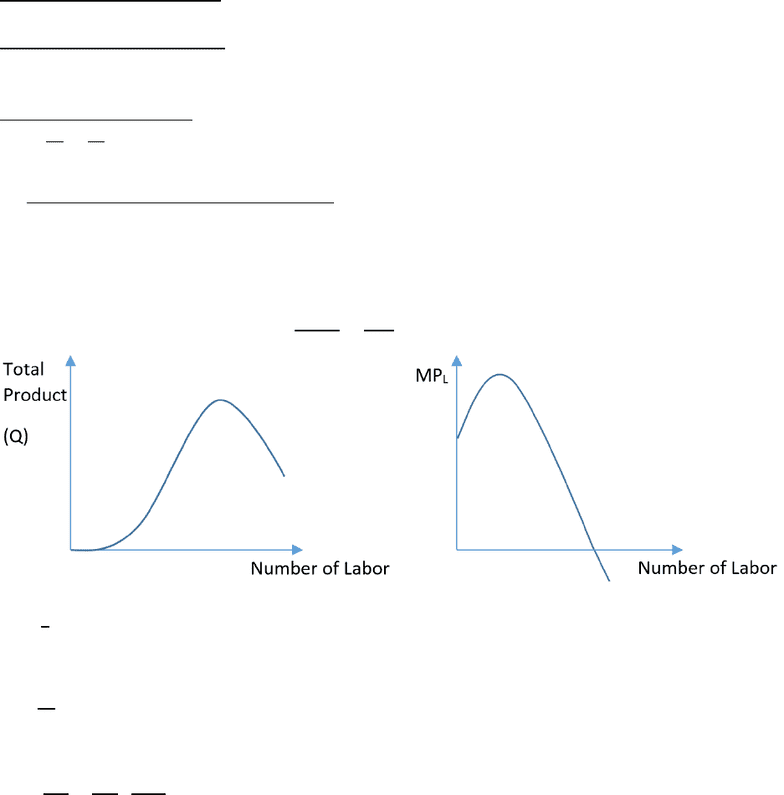# MGEA02H3 Study Guide - Final Guide: 6 Metre

918 views30 pagesUniversity of Toronto Scarborough
MGEA02
Introduction to Microeconomics
Final Exam Guide
PART I Lecture Key Points Review
PART II Exam Questions & Answers and Explanations
OneClass
Unlock document

This preview shows pages 1-3 of the document.
Unlock all 30 pages and 3 million more documents.1. Some basic equations for Production and Productivity:
1) Profit = Total Revenue Total Cost
= TR TC
2) Q = Industry output
q = one firm’s output
Q =
3) Long-run Production function is a relationship between output and inputs.
  
Short-run Production function is a relationship only with number of labors
  
4) Marginal product of labor ( additional output from the additional worker)
MPL = 
 

P.S The Law of Diminishing Marginal Product:
Also called “Law of Diminishing Returns” or “Law of variable Proportions”. The law states that
we will get less and less extra output when we add additional element of an input while holding
other inputs fixed. The marginal product of each unit of input will decline as the amount of that
input increases holding all other inputs constant.


 
5) Average Product of Labor
APL =
6) Average Cost
AC = 
7) Marginal Cost
MC = 
 
 =
 , where TVC = Total Variable Cost
OneClass
Unlock document

This preview shows pages 1-3 of the document.
Unlock all 30 pages and 3 million more documents.8) Production Function:   
Cobb-Douglas Function:   K= Capital, L=Labor
9) Short run = period too short for firms to change production capacity (K is fixed in amount)
Long run = period long enough for firms to change plant capacity, and for new firms to enter
industry (or exit)
Very long run = period long enough for technology to change
10) Total Cost = TC =    , where is the price of per unit capital, is the price per
unit of labor
= (rental) cost of capital equipment
=cost of labor
In the Short-run,
K is fixed, L is variable.
TC =  
= Fixed Cost + Variable Cost
Past Exam Question
A firm in a perfectly competitive constant cost industry produces olives in southern Italy. There are 100
identical firms producing olives in the initial short and long run equilibrium. Total costs in the short run
are given by: TC = q2 + 20q + 256, q ≥ 2 where q is output per day and TC is the total cost per day in
dollars. Each firm has fixed costs of \$192 (already included in the TC equation above). The demand
function for olives is given by P = 100 - .03Q.
a). what is the price of olives in this current short and long run equilibrium?
(A) \$38 (B) \$42 (C) \$46 (D) \$48 (E) \$50
(F) \$52 (G) \$54 (H) \$56 (I) \$58 (J) none of the above
b). What is the lowest price above which firms in this industry will just start making some contribution
towards covering their fixed costs of production in the short run?
(A) \$8 (B) \$22 (C) \$26 (D) \$30 (E) \$36
(F) \$38 (G) \$40 (H) \$42 (I) \$44 (J) none of the above
a) Q = 100q, given P = 100 0.03Q, so we can have
P = 100 0.03Q = 100 0.03 (100q)
P = 100 3q
MC = 
   
Let MC = Demand curve
2q+20 = 100 3q
q = 16
OneClass
Unlock document

This preview shows pages 1-3 of the document.
Unlock all 30 pages and 3 million more documents.